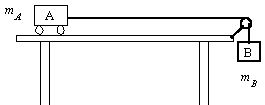Sample Problems from Arnold Arons' Book Homework and Test Questions for Introductory Physics Teaching

Problem 3.40: Consider the situation illustrated here: the system accelerates when object B is released. The inertial effects of both the string and the pulley are negligible. The string is essentially unstretchable.1. How does the acceleration of body A compare with that of body B? Are the accelerations equal or different? If different, which is larger? Explain your reasoning.
2. While the system is accelerating, how does the magnitude of the force exerted by the string on cart A compare with the weight of body B? Is the force exerted by the string on cart A smaller than the weight of body B? (An algebraic solution is not being called for; the reasoning should performed quantitatively.) Explain your reasoning.
3. Suppose that mB is increased while mA remains unchanged. What will happen to the acceleration of the system? Will it increase, decrease, or remain unchanged? What will happen to the force the string exerts on body A? What will happen as mB is increased further and mA becomes very small relative to mB ? Explain your reasoning.
4. Describe what will happen to the acceleration of the system and to the force the string exerts on mA if the changes in part (c) are reversed and mB becomes very small relative to mA. Explain your reasoning.
5. Suppose carts A and B are connected by a rope with significant mass rather than by a massless string. Will the acceleration of the system be uniform or nonuniform? If nonuniform, will the acceleration increase or decrease after release of cart B? Explain your reasoning.
6. What difficulties would be introduced into the problem if the string or rope connecting the two bodies were stretchable rather than unstretchable?
Problem 9.9: Is it possible to connect two identical batteries to make two bulbs in series light just as brightly as one alone across one battery? If so, sketch and explain the circuit diagram. If not, explain your reasoning.

Problem 9.11: You have heard the term "short circuit" used in a variety of circumstances. Define this term operationally. (This means that you must describe actions and observations of results of these actions. Words or synonyms alone are not adequate.)